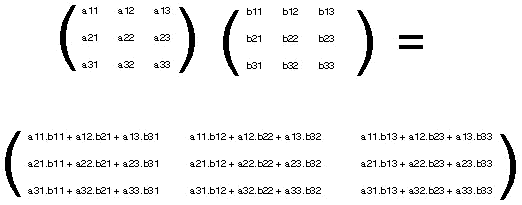## 3x3 matrix multiplication calculator

 Matrix A = Matrix B =
 Result: A x B = A x B =

The 3x3 Matrix Multiplication Calculator to find multiplication between the three matrices A and B.

### 3x3 Matrices Multiplication FormulaThinkcalculator.com provides you helpful and handy calculator resources.